# Modeling Telephony Hybrids using 2 x 2 Matrices

Author: Douglas Rice

Copyright Douglas Rice, doug.h.rice@btinternet.com , 2005,2008,2015

### Further Notes

The theory in this web page draws on information in  and . In 1984 a problem with using an EMC filter inserted in a telphone line prompted me to dig out my University course notes on modelling filters using ABCD parameters. For ABCD parameters see . A search of the local reference library did not come up clear diagrams of the impedance looking into the EMC filter.  explains some of this. Over a large number of evenings, I used my Nascom 2 computer to write some Pascal to calculate the input impedance of the EMC filter.  was a good paper that described how to model Telephone exchanges using ABCD parameters. It was thought too complicated to write Pascal for the equations. The equations for the ABCD model are quite complicated.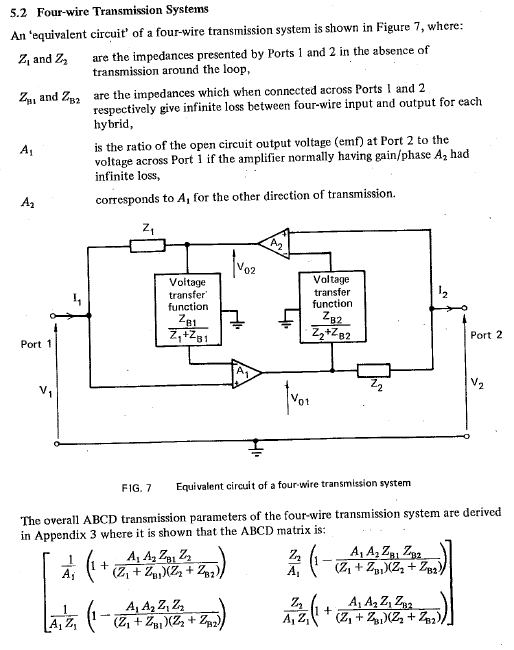(C) permission requested and granted. mar 2017

It occurred to me that I could break the Telephone exchange into three 2x2 matrices, which made the Pascal much simpler.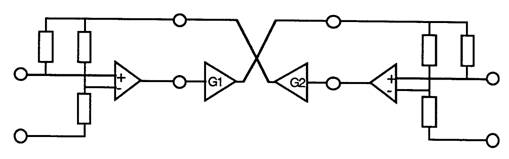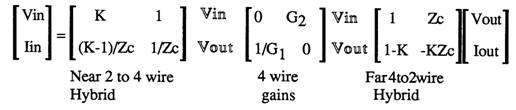With the rise of the Internet, Wikipedia, and search engines, I concluded my use of ABCD parameters did not appear elsewhere. After 21 years, I decided to put my web page on my website. In 2009, I ported the Pascal code to JavaScript. 30 years on it would be of interest to record this. The JavaScript and Flot Graps allows me to animate the graphs for different Telephone line lengths. Things of interest are what is the impeadance looking into the Telephone line. What is the Sidetone for different lines lengths. Sidetone is how much does the Talker hear of their own voice, so the Ration E/M is of interest. What value of =Zb is required. =Zb is my notation for equalZb which is the balance impedance, which is the impedance required to give minimun side tone.

### References

``` P K Webb Post Office Research Department Report 630, Computation of the Characteristics of Telephone Connections,1977.

 R Smith J W Cook, An Integrated Circuit For Analogue Line Interfaces With adaptive Transmission And Extra Facilities, Br Telecom Technology J Vol 5 No 1 January 1987, setion 4 page 36-37.

 D V Mercy, Phase From Amplitude, Electronics and Wireless World, June 1988 Vol 94 Number 1628.

 D.V Mercy, Amplitude From Phase, Electronics and Wireless World, July 1988 Vol 94 Number 1629..

 BP0 603

 D.L Richards, Telecommunication By Speech, Butterworths,1973

 F.F. Kuo, Network Analysis and Synthesis, Wiley International,1962

 N.A Pashtoon,Chap 4 of D.F.EIIiott, Handbook of Digital Signal Processing Engineering Applications,Academic Press, 1987

 Course notes - Sheffield University

  https://en.wikipedia.org/wiki/Two-port_network

 http://www.electrical4u.com/abcd-parameters-of-transmission-line/

 Flot -  FLOT charts

  BT's SIN 351 Issue 4.6,April 2014 and 352  ( 08/12/2015 )

  BT's SIN site for SIN 351 Issue 4.6,April 2014 and 352

```

### Examples - Phone + Artificial Line + Termination. Still in update

In real life Phone use balanced signals, these models are unbalanced, but are still valid. publishes two terminations, but not =Zb.

The Test Circuit: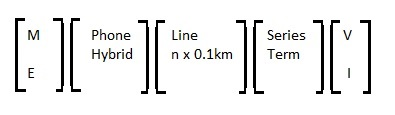converted to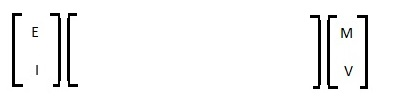V is a function of M. Using the Series Termination and short V can be made to be 0.

Use Shunt Termination when you need Io =0 and Vo != 0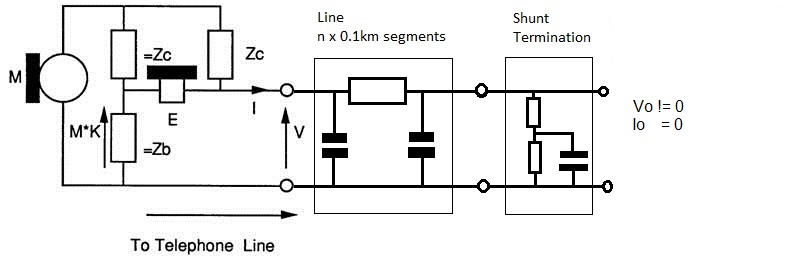Use Series Termination when you need Vo =0 and Io != 0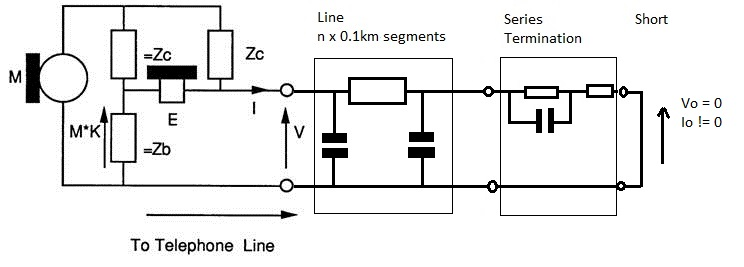a_artline Impedance looking into the Artifical line presented to Phone.

a_artline4W.htm Side Tone Loss. E/M for circuit above. Note Zb is not specified in 

a_artline4Wnew.htm Side Tone Loss. E/M for circuit above. Note Zb is not specified in 

a_artlineZb.htm Calculating Zterm for zero Side tone. Not really a useful thing to know.

a_424L424.htm

### Other notes

Alternative circuits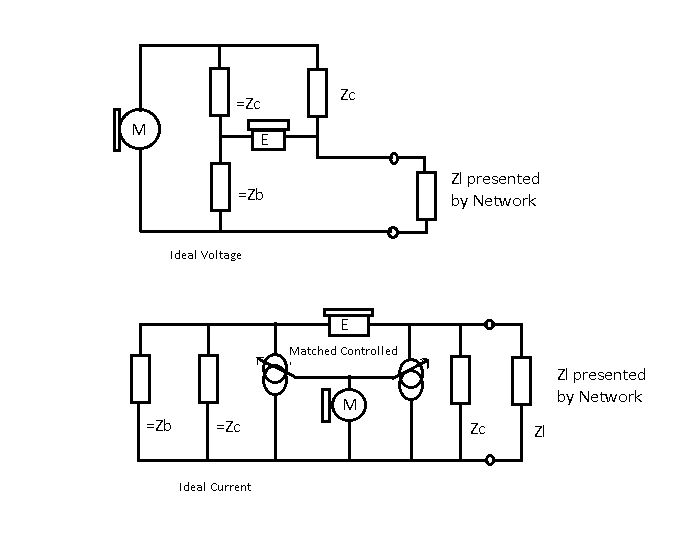### Terminal Impedance

See SIN351 3.4.1. Terminal Input Impedance

The impedance necessary to satisfactorily terminate the BT network interface at the NTP so as to prevent instability is represented by the three-element network shown in Figure 1. A minimum return loss of 12 dB should be achieved by terminal equipment against the three-element network of Figure 1.

```--[370R]--+--[620R]--+--
|          |
+----||----+
310nF
```

### Network Impedance

See SIN351 3.4.2. Network Input Impedance The input impedance of the network at the NTP is represented by a range of impedances comprising the input impedance of the local exchange, modified by the impedance of a random variety of local cable types/characteristics. The nominal exchange input impedance is 300R + 1000R || 220 nF

```--[300R]--+--[1000R]--+--
|          |
+----||----+
220nF
```

This may be regarded as the appropriate value for use by terminal designers. NOTE: It can be shown that this is about Zterm + 2km of artificial line, using a_artline Impedance looking into the Artifical line presented to Phone.

### Zc,Zb and SIN351

My paper was written 30 years ago, and well before SIN351.

My paper writes about Zc, the Input impedance and output Impedance for the Hybrid.See

The Balance impedance is the impedance that, connected across the terminals, would give zero side tone.

The Phone circuit is like a Wheatstone bridge. The Microphone voltage M drives the bridge and the Earphone is the detector.

When Zb/(Zc+Zb) == (=Zc/(=Zc+=Zb)), the voltage across E is zero when M in not zero.

When Zc/Zb == (=Zc/=Zb), the voltage across E is zero when M in not zero.

I used the notation Zb for the Balance impedance and I used =Zc and =Zb to mean equalZc and equalZb.

The Terminal impedance, Zterm is 370R+620R/310nF

The Network impedance, Zexch is 300R+1000R/220nF

If you use my webpage: a_artline , it can be set up to show that Zexch is about equal to 2Km of artifical line terminated bt Zterm.

```So, when Line length is 2km :

Zc = Zterm

Zb = Zexch

As To get minimal sidetone, set Zb to equal Zexch which is approximatly equal to 2Km of line terminated by Zterm.

My code has:
//The terminal Figure 1: Terminal Complex Impedance Network = 370+ 620//310nf
var z_complex_z = z_abc(370, 620, 310.0e-9 , freq[c] );

//The nominal exchange input impedance is 300 Ù + 1000 Ù || 220 nF (see Figure 2).
var z_complex_znom = z_abc(300.0, 1000.0, 220.0e-9 , freq[c] );

```

### Artificial Line - speeding up the calculations.

Artifical Line is modelled using n x 0.1km sections. The range of local lines can be represented by between 0 km and 9 km of 0.5 mm copper cable with nominal characteristics of 168 Ohm/km and 50 nF/km (attenuation at 1600 Hz of 1.7 dB/km).

```    100 metre section = O---[===]----O
I       I
===     ===  2.5nF
I       I
O---[===]----O
16.8 ohm
```

Artifical Line is modelled by n x 0.1km sections. For 7.5km of artifical line, append 75 segments of line, this requires 75 2x2 matrix multiplications using complex numbers. On My Nascom2 this took a very long time and rounding errors on 32bit floats was considered a problem. ( See  and  )

Using the word append gets around getting muddled with "Add" and "Multiply".

We add line segments by multiplying 2x2 matrices.

We add components by multiplying 2x2 matrices. see  and 

I take liberties with notation due to using ascii text using [ ] for matrix. [seg] or seg[] means 2x2 matrix. Other people use capital letters.

```seg[] = [C][R][C]

[C] is:

[Vin] = [ 1     0  ] [Vo]
[Vi ]   [ 1/jwC 1  ] [Io]

w=2*Pi*freq, where Freq is in Hz.

[R] is:

[Vin] = [ 1   R  ] [Vo]
[Vi ]   [ 0   1	 ] [Io]

```

The method used here takes the segments of line, doubles the length and conditionally appends this to the end to end calculation.

This cuts down the number of matrix multiplications required.

```//length is length of artificial line in km.
we model it in 0.1 sections.

a[] = 0.1km section
len = len * 10
while length > 0 {
if ( LSB of Len equals 1 ) {
append a[] onto end to end chain.
apend a[] to itself to get 0.2km section
len len div 2
}
}
```
```//PROCEDURE lines (length : REAL);
function lines( lineLength ) {

/*
This procedure evaluates line sections for unloaded line.
The model is that of BTNR 1050
Operation is akin to long multipliction , 100 meter sections
*/

var len  = (lineLength * 10.0)&-1;        //{length is km of line}

if ( len != 0 ) { // BEGIN
il=0;
il2=1;

// multz() leaves result in r[]
// multy() leaves result in r[]
// copy r[] into a[]
aar=rar;   aai=rai;
abr=rbr;   abi=rbi;
acr=rcr;   aci=rci;
aqr=rdr;   aqi=rdi;

//init r[] = 1.0
rar=1;      rai=0;
rbr=0;      rbi=0;
rcr=0;      rci=0;
rdr=1;      rdi=0;
/*
16.8/2 ohm
100 metre section = O---[===]----O
I       I
===     ===  2.5nF
I       I
O---[===]----O
16.8/2 ohm
*/
// r[] = identity matrix
multy(0,w*2.5E-9);
multz(16.8,0);
multy(0,w*2.5E-9);
// r[] now has 0.1km section

while ( len > 0 ) {
// Starting at the Least significant bit of line length, conditionally add a section of line.
len = len & -1;
/*
{ original Pascal used. It used a way to get teh LSB by using integer DIV }
WHILE len > 0 DO
BEGIN
IF (len-2*(len DIV 2))=1 THEN
BEGIN

*/
// JAVA script ands 1 with len to get LSB
if ( ( len & 1 ) != 0 ) {

// conditionally add line segment to a[]
ar=aar;  ai=aai;
br=abr;  bi=abi;
cr=acr;  ci=aci;
dr=aqr;  di=aqi;

// a[] contains
aar=ar*rar-ai*rai+br*rcr-bi*rci;
aai=ar*rai+ai*rar+br*rci+bi*rcr;

abr=ar*rbr-ai*rbi+br*rdr-bi*rdi;
abi=ar*rbi+ai*rbr+br*rdi+bi*rdr;

acr=cr*rar-ci*rai+dr*rcr-di*rci;
aci=cr*rai+ci*rar+dr*rci+di*rcr;

aqr=cr*rbr-ci*rbi+dr*rdr-di*rdi;
aqi=cr*rbi+ci*rbr+dr*rdi+di*rdr;

il=il+il2
}

//r[] = r[]+r[]
multmat (rar,rai,rbr,rbi,rcr,rci,rdr,rdi);
// printABCD();
il2=il2+il2;
len= ( len / 2)  ;
}
// copy a[] back into r[]
rar=aar;   rai=aai;
rbr=abr;   rbi=abi;
rcr=acr;   rci=aci;
rdr=aqr;   rdi=aqi;
}
} //END; {line}
```

### ABCD Matrix - summary

```/*
2x2 matrix - ascii text notation.

[Vi]=[A B][Vo]
[Ii] [C D][Io]
*/
```

### Complex Numbers - summary

```multiply:
/*
(ar+jai)(br+jbi) =  (ar*br-ai*bi) +j(ar*bi+ai*br)

inverse:
1/(br+jbi) =    (br-jbi)
--------------------
((br+jbi)*(br-jbi))

1/(br+jbi) =    (br-jbi)
--------------------
(  (br*br+bi*bi)  +j(br*bi-bi*br) )

1/(br+jbi) =    (br-jbi)
--------------------
(  (br*br+bi*bi)  )
*/
```